﻿ 20-sim webhelp > Toolboxes > Animation Toolbox > 3D Animation > Properties > Texture

# Texture

Using the Texture attribute, you can wrap a bitmap file around an object. Using the browse button, you can easily select the desired bitmap file (bmp, jpg, gif, tif, etc.). Using the transparency option you can select a color in the bitmap that should be transparent.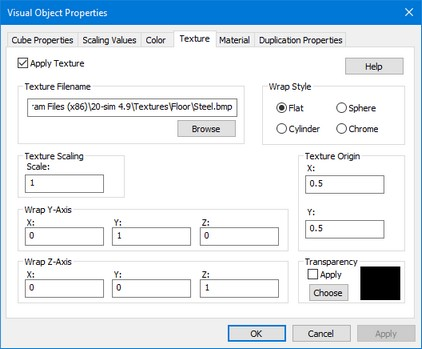## Texture Origin

A texture is defined in generalized coordinates (u,v). The bottom right of the texture has coordinates (0,0) and the top left (1,1). The offset parameters define how much offset is used to project the bitmap. This is shown in the figure below. At the left an offset of (0,0) is shown and at the right an offset (0.2,0.2) is shown.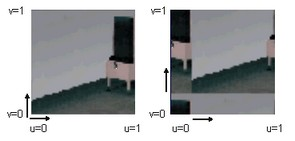Depending on the type of texture wrapping (Flat, Cylinder, Sphere or Chrome) the three wrapping vectors () have a different meaning. This is explained in the next sections. To facilitate the explanation in the pictures below the origin vector equals (0,0,0), the Y-vector equals (0,1,0) and the Z-vector equals (0,0,1).

## Vectors

Using three vectors (Origin, Y-Axis and Z-Axis) and Offset parameters (X, Y) you can define how the texture should be wrapped around an object. The vectors are defined with reference to the object attached reference frame. The object attached reference frame can be made visible by selecting Show Frame. The vectors use generalized coordinates. I.e. the length, width and height of the object are defined as 1 and the vectors are scaled accordingly.

## Flat

The flat wrap conforms to the faces of an object as if the texture were a piece of rubber that was stretched over the object. For a flat texture wrap, the effects of the various vectors are shown in the following illustration.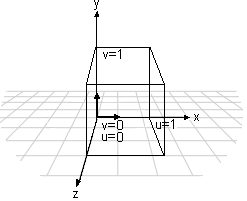• The Origin vector defines the base from which the texture wrapping is performed.
 • The Y-vector equals the v axis of the texture wrap.
 • The Z-vector is always perpendicular to the texture wrap.

## Cylindrical

The cylindrical wrap treats the texture as if it were a piece of paper that is wrapped around a cylinder so that the left edge is joined to the right edge. The object is then placed in the middle of the cylinder and the texture is deformed inward onto the surface of the object. For a cylindrical texture wrap, the effects of the various vectors are shown in the following illustration.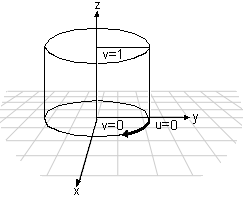• The Origin vector defines the base from which the texture wrapping is performed.
 • The Y-vector specifies the point on the outside of the cylinder where u equals 0.
 • The Z-vector specifies the axis of the cylinder.

## Spherical

For a spherical wrap, the u-coordinate is derived from the angle that the vector [x y 0] makes with the x-axis (as in the cylindrical map) and the v-coordinate from the angle that the vector [x y z] makes with the z-axis. Note that this mapping causes distortion of the texture at the z-axis.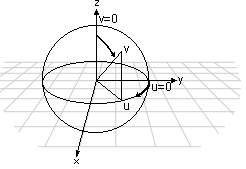• The Origin vector defines the base from which the texture wrapping is performed.
 • The Y-vector specifies the point on the outside of the sphere where u equals 0.
 • The Z-vector specifies the point on the outside of the sphere where v equals 0.

## Chrome

A chrome wrap allocates texture coordinates so that the texture appears to be reflected onto the objects. The chrome wrap takes the reference frame position and uses the vertex normals in the mesh to calculate reflected vectors. The texture u- and v-coordinates are then calculated from the intersection of these reflected vectors with an imaginary sphere that surrounds the mesh. This gives the effect of the mesh reflecting whatever is wrapped on the sphere.